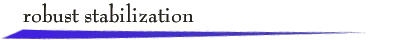Formulation

Suppose a nominal plant model has the strictly-proper, rational transfer function P(s). Due to various modeling simplifications or errors, we adopt a plant uncertainty model. Corresponding to a stable frequency weighting function W(s), the family of uncertain plants is defined by

PD (s) = [ 1 + D (s) W(s)] P(s)

The uncertainty parameter D(s) is any stable transfer function satisfying | D (jw) | £ 1 for all w ³ 0, and such that any unstable pole of P(s) is also a pole of PD (s).

Consider the design of a compensator C(s) in a unity feedback system to stabilize the family of uncertain plants. For any plant PD(s) in the family, the closed-loop system transfer function is

```        C(s)PD(s)
TD(s) = --------------
1 + C(s)PD(s)
```

Theorem

The compensator C(s) is such that the family of closed-loop transfer functions TD(s) is stable if and only if the following two conditions hold:

1. Nominal Stability: The nominal closed-loop transfer function

```        C(s)P(s)
T(s) = --------------
1 + C(s)P(s)
```
is stable.

2. Small Gain: For all w ³ 0, |W(jw) T(jw)| < 1

Applet

The robust stabilization applet comprises two parts: formulation of the plant uncertainty model, and design of the compensator. You first enter the nominal plant model

```        cmsm + ... + c1s + c0
P(s) = -----------------------
sn + an-1sn-1 + ... + a0
```

where 0 £ m < n £ 3, in terms of numerator and denominator coefficients. This P(s) is displayed on the first set of Bode plots. Next choose a suitable frequency weighting function

```        k (s - z1)(s - z2)
W(s) = -------------------
(s - p1)(s - p2)
```

by dragging the poles and zeros with the mouse. The corresponding uncertain plant family PD (s) is shown by shading on the open-loop Bode plots.

To design a compensator that will stabilize the uncertain plant family, select a compensator C(s) by dragging the poles and zeros on the compensator Bode plot with the mouse to satisfy the Small Gain Condition as displayed on the Bode magnitude plot for | W(jw)T(jw) |. For example, to explore a constant gain compensator, C(s) = K, place the compensator zeros on top of the poles, and change the gain by dragging vertically. Nominal Stability will be indicated automatically. Of course, depending on the weighting function W(s) and form of compensator C(s) chosen, robust stabilization might not be possible.return to demonstrations page

 Applet by Steven Crutchfield